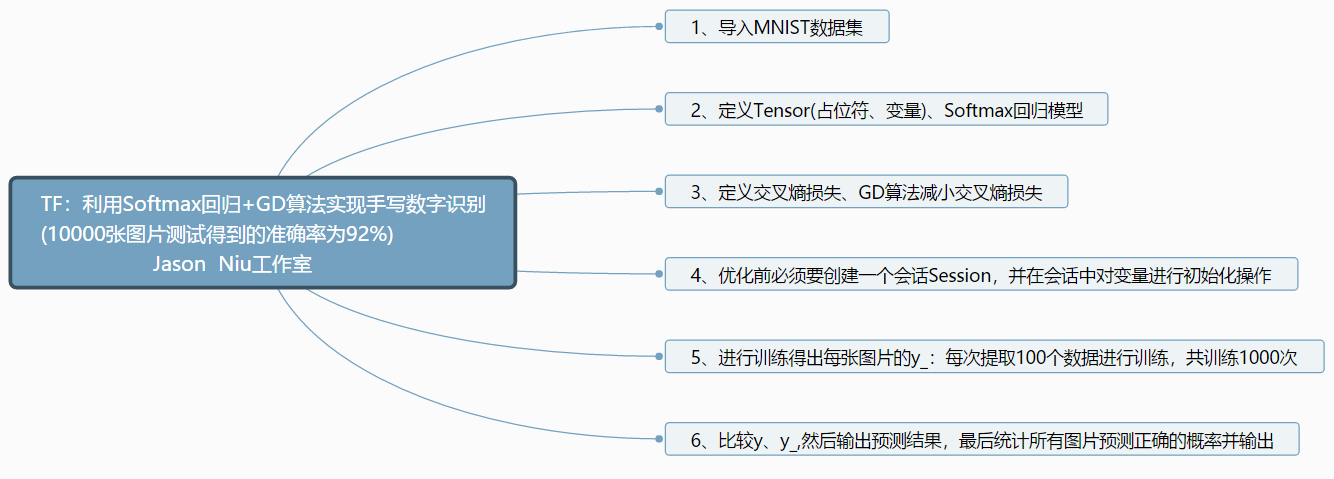•  * 1-1000010000个数乱序的出来，但是其中有个数字写错了（可能重复，或者不是1-10000中的数字），如何快速找出？  */ package com.java.demo.algorithm; public class FindNum {  /**  * @param ...
/**
* 1-10000这10000个数乱序的写出来，但是其中有个数字写错了（可能重复，或者不是1-10000中的数字），如何快速找出？
*/
package com.java.demo.algorithm;
public class FindNum {
/**
* @param args
*/
public static void main(String[] args) {
int[] array = {1,3,2,4,5,6,10,9,8,1};
System.out.println(findTheErrorNum(array));
}
private static String findTheErrorNum(int[] dest){
int length = dest.length;
int[] array = new int[length];
for (int i = 0; i < length; i++) {
int k = dest[i];
if (k <= 0 || k > length) {
//因为前提就是数组中的数字是1-10000，因此当数字小于等于0，或者大于10000时就是错误的数字，直接返回
return String.valueOf(k);
}
if (array[k-1] == 0) {
array[k-1] = k;
} else {
//array中第k个数已经存上值时，当前k是重复的数字，返回
return String.valueOf(k);
}
}
return "未发现错误的数字！";
}
}
展开全文string class
• 1-10000之间的对称数 知识普及一哈：一个整数，它的各位数字如果是左右对称的，则称这个数是对称数。例如：1234321、123321等。 对称数也叫做回文数。 一般来说，位数大于或等于两位。最小的对称数是11，没有...
写出1-10000之间的对称数
知识普及一哈：一个整数，它的各位数字如果是左右对称的，则称这个数是对称数。例如：1234321、123321等。 对称数也叫做回文数。 一般来说，位数大于或等于两位。最小的对称数是11，没有最大的对称数，因为数位是无穷的。
写运算函数
	//判断是否是对称数
let isSymmetry = function(num) {
let sNum = num.toString();
let slength = sNum.length;
for (let i = 0; i < slength / 2; i++) {
if (sNum.charAt(i) !== sNum.charAt(slength - 1 - i) || slength < 2) {
return false;
}
}
return true;
}

或者这样

//对称数的逆序数等于自身
let isSymmetry = function(num) {
nInversionNumber = +(num.toString().split("").reverse().join(""));
if (num === nInversionNumber && num >= 10) {
return true;
}
return false;
}


这样使用，最终拿到一个对称数的数组集合

//1-number之间的对数
let arr = [],number = 10000;
for (let j = 0; j < number; j++) {
isSymmetry(j) ? arr.push(j) : false
}

console.log(arr, '返回对数数组')

还有其他的计算写法，但是出于代码量及运行性能考虑，推荐使用这两种之一。


展开全文js笔试题 js面试题
• TF：利用是Softmax回归+GD算法实现MNIST手写数字图片识别(10000张图片测试得到的准确率为92%) 目录 设计思路 全部代码 设计思路 全部代码 #TF：利用是Softmax回归+GD算法实现手写数字识别(10000...
TF：利用是Softmax回归+GD算法实现MNIST手写数字图片识别(10000张图片测试得到的准确率为92%)

目录

设计思路

全部代码

设计思路全部代码

#TF：利用是Softmax回归+GD算法实现手写数字识别(10000张图片测试得到的准确率为92%)
#思路：对输入的图像，计算它属于每个类别的概率，找出最大概率即为预测值
import tensorflow as tf
from tensorflow.examples.tutorials.mnist import input_data

x = tf.placeholder(tf.float32, [None, 784])
W = tf.Variable(tf.zeros([784, 10]))
b = tf.Variable(tf.zeros())
y = tf.nn.softmax(tf.matmul(x, W) + b)
y_ = tf.placeholder(tf.float32, [None, 10])

cross_entropy = tf.reduce_mean(-tf.reduce_sum(y_ * tf.log(y)))

sess = tf.InteractiveSession()
tf.global_variables_initializer().run()
print('start training...')

for _ in range(1000):
batch_xs, batch_ys = mnist.train.next_batch(100)
sess.run(train_step, feed_dict={x: batch_xs, y_: batch_ys})

correct_prediction = tf.equal(tf.argmax(y, 1), tf.argmax(y_, 1))
accuracy = tf.reduce_mean(tf.cast(correct_prediction, tf.float32))

print(sess.run(accuracy, feed_dict={x: mnist.test.images, y_: mnist.test.labels}))  # 0.9185

相关文章

TF：利用是Softmax回归+GD算法实现MNIST手写数字识别(10000张图片测试得到的准确率为92%)

展开全文• TF：基于CNN(2+1)实现MNIST手写数字图片识别准确率提高99% 导读 与Softmax回归模型相比，使用两层卷积的神经网络模型借助了卷积的威力，准确率高非常大的提升。 目录 输出结果 代码实现 输出结果 ...
TF：基于CNN(2+1)实现MNIST手写数字图片识别准确率提高到99%

导读
与Softmax回归模型相比，使用两层卷积的神经网络模型借助了卷积的威力，准确率高非常大的提升。

目录

输出结果

代码实现

输出结果

Extracting MNIST_data/train-images-idx3-ubyte.gz
Extracting MNIST_data/train-labels-idx1-ubyte.gz
Extracting MNIST_data/t10k-images-idx3-ubyte.gz
Extracting MNIST_data/t10k-labels-idx1-ubyte.gz

step 0, training accuracy 0.1
step 1000, training accuracy 0.98
step 2000, training accuracy 0.96
step 3000, training accuracy 1
step 4000, training accuracy 1
step 5000, training accuracy 0.98
step 6000, training accuracy 0.98
step 7000, training accuracy 1
step 8000, training accuracy 1
step 9000, training accuracy 1
step 10000, training accuracy 1
step 11000, training accuracy 1
step 12000, training accuracy 1
step 13000, training accuracy 0.98
step 14000, training accuracy 1
step 15000, training accuracy 1
step 16000, training accuracy 1
step 17000, training accuracy 1
step 18000, training accuracy 1
step 19000, training accuracy 1

代码实现

#TF：基于CNN实现MNIST手写数字识别准确率提高到99%

import tensorflow as tf
from tensorflow.examples.tutorials.mnist import input_data

……

if __name__ == '__main__':
x = tf.placeholder(tf.float32, [None, 784])
y_ = tf.placeholder(tf.float32, [None, 10])
x_image = tf.reshape(x, [-1, 28, 28, 1])  #x_image就是输入的训练图像

W_conv1 = weight_variable([5, 5, 1, 32])
b_conv1 = bias_variable()
h_conv1 = tf.nn.relu(conv2d(x_image, W_conv1) + b_conv1)  #是真正进行卷积计算，再选用ReLU作为激活函数
h_pool1 = max_pool_2x2(h_conv1)  #调用函数max_pool_2x2 进行一次池化操作。

W_conv2 = weight_variable([5, 5, 32, 64])
b_conv2 = bias_variable()
h_conv2 = tf.nn.relu(conv2d(h_pool1, W_conv2) + b_conv2)
h_pool2 = max_pool_2x2(h_conv2)

W_fc1 = weight_variable([7 * 7 * 64, 1024])
b_fc1 = bias_variable()
h_pool2_flat = tf.reshape(h_pool2, [-1, 7 * 7 * 64])
h_fc1 = tf.nn.relu(tf.matmul(h_pool2_flat, W_fc1) + b_fc1)
keep_prob = tf.placeholder(tf.float32)
h_fc1_drop = tf.nn.dropout(h_fc1, keep_prob)

W_fc2 = weight_variable([1024, 10])
b_fc2 = bias_variable()
y_conv = tf.matmul(h_fc1_drop, W_fc2) + b_fc2

cross_entropy = tf.reduce_mean(
tf.nn.softmax_cross_entropy_with_logits(labels=y_, logits=y_conv))

correct_prediction = tf.equal(tf.argmax(y_conv, 1), tf.argmax(y_, 1))
accuracy = tf.reduce_mean(tf.cast(correct_prediction, tf.float32))
sess = tf.InteractiveSession()
sess.run(tf.global_variables_initializer())

for i in range(20000):  # 训练20000步
batch = mnist.train.next_batch(50)
# 每100步报告一次在验证集上的准确度
if i % 100 == 0:
train_accuracy = accuracy.eval(feed_dict={
x: batch, y_: batch, keep_prob: 1.0})
print("step %d, training accuracy %g" % (i, train_accuracy))
train_step.run(feed_dict={x: batch, y_: batch, keep_prob: 0.5})
print("test accuracy %g" % accuracy.eval(feed_dict={
x: mnist.test.images, y_: mnist.test.labels, keep_prob: 1.0}))


相关文章
TF：基于CNN(2+1)实现MNIST手写数字识别准确率提高到99%


展开全文• 本文使用Tensorflow框架进行Python编程实现基于卷积神经网络的手写数字识别算法，并将其封装在一个GUI界面中，最终，设计并实现了一个手写数字识别系统。机器学习 python Tensorflow 图像识别
• ## 基于SVM技术的手写数字识别

万次阅读 多人点赞 2019-07-29 22:51:39
老师常说，在人工智能未发展起来之前，SVM技术是一统江湖的...1.手写数字识别技术的含义 2.手写数字识别技术的理论价值 3.数字识别技术的难点 二、SVM技术 1.SVM方法简介 2.线性可划分问题 3.近似线性可分问题...python 计算机视觉 SVM PyQt
•  Mnist手写数字识别是Kaggle上一个很经典的机器学习数据集，里边包括55000张训练数据和10000张图片的测试数据，每张图片大小为28*28像素的单通图片。该任务为通过机器学习来识别图片中的数字属于0，1，2，3，4，...神经网络 Tensorflow
• 当学习一门新的编程语言的时候，我们总是以输出“hello word”作为学习这门编程语言的开始，表示我们开启...MNIST:在实现手写数字识别需要使用手写数字的图片，是MNIST下载的。MNIST(Modified National Institute o
• ## caffe识别手写数字

千次阅读 2016-10-11 16:52:23
网上很多教程指导建立caffe中的mnist手写数字识别模型（比如http://www.cnblogs.com/aaa-YK/p/5537329.html），运行后可以得到一个分类文件lenet_iter_10000.caffemodel。  做为一个linux+python小白，尝试使用...
• import torch ... print('Accuracy of the network on the 10000 test images: {} %'.format(100 * correct / total)) # Save the model checkpoint torch.save(model.state_dict(), 'model.ckpt')python
• public class Test02 { public static void main(String[] args) { // TODO Auto-generated method ...\\d{1,4}(\\.\\d{2})?"; System.out.println("9999.99".matches(er)); System.out.pr...正则表达式
• 手写数字识别目前研究的最新成果，以及所用的数据集等综述MNIST 深度学习 python
• 10000 , 784 ).astype( 'float32' ) x_train /= 255 x_test /= 255 print(x_train.shape[ 0 ], 'train samples' ) print(x_test.shape[ 0 ], 'test samples' ) # convert class vectors to binary class ...神经网络 keras
• 手写数字识别通常作为第一个深度学习在计算机视觉方面应用的示例，Mnist数据集在这当中也被广泛采用，可用于进行训练及模型性能测试； 模型的输入为： 32*32的手写字体图片，这些手写字体包含0~9数字，也就是相当于...
• 手写数字识别是机器学习领域中的一个经典应用，很多机器学习算法以这个问题作为示例，其地位相当于程序界的hello world。这个问题具有以下两个特点： 问题复杂度适中。手写识别是一门很深的学问，但这里将问题域...人工智能
• 本文演示了利用TensorFlow进行基于Softmax的手写数字识别的例子，这原本是其文档中的一个示例（数据集采用MINST）参作为学习TensorFlow的一个实例教程这是一个非常不错的选择。同时，借由这个例子也可以方便读者理解...Softmax TensorFlow 深度学习
• ## 手写数字的分割和识别

千次阅读 多人点赞 2019-04-08 11:41:23
在机器学习领域，手写数字数据集MNIST之于机器学习几乎相当于HelloWorld之于编程语言，其重要地位不言而已。但是，然后呢？给你一张如下所示的图片，你的模型能否也预测出结果？（其实下面这个应用就是OCR领域的内容...字符分割 opencv keras 深度学习
• 上一节我们自己代码训练了只有一个神经元的反相器，它虽然只有一点点代码，但却让我们加深了梯度下降算法和反向传播...在训练处反相器以后，我开始大胆的尝试自己一个全连接的神经网络，来训练手写数字。这并不难，java 深度学习 神经网络 算法
• SVM简介 知乎上的一个回答我认为是史上...开发环境 Windows10 + VS2013 + Qt580 + OpenCV300主要代码 利用opencv-SVM算法和Mnist数据集封装成一个单例模式的数字识别检测器。DigitsDetector.h#ifndef DIGITSDETEC...SVM opencv 支持向量机 机器学习
• # 键盘上输入一个正整数n，找出1000至10000之间所有各位数字之和等于n的数 # 如输入32,则有9869满足要求。因为9869各位上数字之和等于32 用python解决，需要详细的代码和解释，谢谢python python
• 最近的学习项目中需要一个数字从0慢慢增加指定数字，然后想了好久才做出来。一开始是想用循环做，循环里面用delay(),但是发现不太好用（可能是我不会用），然后想用循环里面套setTimeout，后来发现不行，一番百度...
• 引言 在学习了深度学习的全连接网络后，我们 用Keras实现手写数字识别。 数据集 采用的数据集是基本教程...如下是数字1的一个例子,我们的目的是做出一个模型，将这784个数值输入这个模型，然后它的输出是1。 使用...神经网络
• 手写识别的应用场景有很多，智能手机、掌上电脑的信息工具的普及，手写文字输入，机器识别...我们来尝试搭建下手识别中最基础的手写数字识别，与手写识别的不同是数字识别只需要识别0-9的数字，样本数据集也只需...深度学习 tensorflow python
• 誓做堂主司徒 ... 先看看以下两个有关大写数字的表格： 1、数码与大、小写数字的对照表： 数码 小写数字 大写数字 0 ○ 零 1 一 壹 2 二 贰 3 三 叁/参 4 四 肆 5 五 伍 6 六 陆 7 七 柒 8 八 捌
• 神经网络实现迷宫游戏的思路，在本篇当中也如何使用神经网络实现迷宫的，但是研究了一下， 感觉有些麻烦不太好弄，所以就选择了比较常见的方式，实现手写数字识别（所谓的MNIST）。 二、人工神经网络简介 ...神经网络 c++ 算法
• 1、xgboost 安装安装问题这里就不再做赘述，可参考前面的博文： http://blog.csdn.net/eddy_zheng/article/details/501845632、手写数字识别这里先说明下，xgboost用作手写字符的分类效果并不是最好的，这里仅仅...机器学习 xgboost
• ## 神经网络之手写数字识别

千次阅读 多人点赞 2021-05-05 19:28:57
神经网络之手写数字 文章目录神经网络之手写数字00. 在之前01. 代码框架02. 开始做一些准备工作03. 框架的开始04. 训练模型构建05. 手写数字的识别06. 想看源码的同学戳这里07. 思考 首先鼓掌，又是一个有收获的...
• 此项目为三层神经网络识别，激活函数采用sigmoid函数，数据集为mnist手写数字集，训练集包括60000个样本，测试集10000个样本。 第一部分,创建神将网络模型 import numpy import matplotlib.pyplot #%matplotlib ...
• 手写数字识别是每个学习神经网络的人上手操作的必由之路，今天在前人肩膀上做了些小小的尝试。 话不多说，开始~ 1.导入相关模块数据包（需提前配置tenseorflow模块） 在tenseorflow模块中内置了MNIST数据集，其中...机器学习 BP神经网络 人工智能...